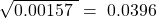Probability Concepts | IFT World
IFT Notes for Level I CFA® Program
IFT Notes for Level I CFA® Program

# Part 3

## 4. Portfolio Expected Return and Variance of Return

### Expected Return

A portfolio’s expected return can be calculated as: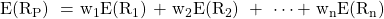where:

wn = portfolio weight of nth security in the portfolio

Rn = expected return of nth security in the portfolio

n = number of securities in the portfolio

We will discuss portfolio expected return and variance of return using a two-stock portfolio.

Example

40% of the portfolio is invested in Stock A and 60% is invested in Stock B. As shown in the table below, the expected return of each stock depends on the economic scenario.

 Scenario P(Scenario) Expected returns of A Expected returns of B Recession 0.25 2% 4% Normal 0.50 8% 10% Boom 0.25 12% 16%

This information can also be presented as a joint probability function of A’s and B’s returns:

 RB = 4% RB = 10% RB = 16% RA = 2% 0.25 0 0 RA = 8% 0 0.50 0 RA = 12% 0 0 0.25

Row 1 and Column 1 represent the returns of A and B respectively. The other cells contain probabilities. Calculate the expected return of A and B.

Solution:

Given the data presented above:

The expected return of A is: 0.25 x 2 + 0.50 x 8 + 0.25 x 12 = 7.5%.

The expected return of B is: 0.25 x 4 + 0.50 x 10 + 0.25 x 16 = 10%.

Expected return of the portfolio = weight of A in the portfolio x expected return of A + weight of stock B in the portfolio x expected return of B = 0.4 x 7.5 + 0.6 x 10 = 9%

The expected portfolio return is 9%. As the term implies, this is the expected return. The actual return will vary around 9%. The amount of variability is measured by the variance.  In order to determine the variance of return, we must first calculate the covariance.

### Covariance

Covariance tells us how movements in a random variable vary with movements in another random variable, whereas variance tells us how a random variable varies with itself. Assume there are two random variables Ri and Rj. The forward-looking, population covariance between Ri and Rj (used to measure how they move together) is given by: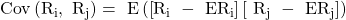where:

ERi = expected return for variable Ri

ERj = expected return for variable Rj

If the random variables are returns, the units of both forward-looking covariance and historical variance would be returns squared.

The sample covariance between two random variables Ri and Rj is the average value of the product of the deviations of observations on two random variables from their sample means. It is calculated as follows: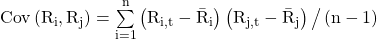Unlike the population covariance, the sample covariance is based on historical data set. This reading focuses on covariance in a forward-looking sense.

Example

Continuing with our previous example, calculate the covariance of returns between A and B.

Solution:

Say Ri represents the return on A and Rj represents the return on B, we have already calculated the expected returns of A and B as 7.5% and 10% respectively. The covariance of returns is:

E [(Ri – 7.5) (Rj – 10)]

= 0.25(2% – 7.5%)(4% – 10%) + 0.5(8% – 7.5%)(10% – 10%) + 0.25(12% – 7.5%)(16% – 10%)

= 0.000825 + 0 + 0.000675 = 0.0015

### Correlation

The problem with covariance is that it can vary from negative infinity to positive infinity which makes it difficult to interpret. To address this problem, we use another measure called correlation. Correlation is a standardized measure of the linear relationship between two variables with values ranging between -1 and +1.

• A correlation of 0 (uncorrelated variables) indicates an absence of any linear (straight-line) relationship between the variables.
• A correlation of +1 indicates a perfect positive relationship.
• A correlation of -1 indicates a perfect negative relationship.

It is computed as:

ρ(Ri,Rj) = Cov (Ri,Rj) / [σ(Ri)  σ(Rj)]

The above correlation measure is forward-looking as it is calculated by dividing the forward-looking covariance by the product of forward-looking standard deviations.

Historical or sample correlation = Historical or sample covariance between two variables / (Sample standard deviation of variable Ri * Sample standard deviation of variable Rj)

We will now apply this formula to calculate the correlation between the returns of A and B from our example. We have already shown that the covariance of returns is 0.0015. In order to calculate the correlation, we need the standard deviation of A and B. Using a financial calculator, we can determine that the standard deviation of A is 0.0357 and the standard deviation of B is 0.0424. The correlation,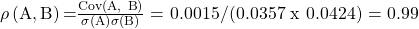.

The correlation of 0.99 (almost 1) implies a very strong positive relationship between the returns of A and B. This is more meaningful than the covariance number of 0.0015 which tells us that there is a positive relationship between the returns of A and B but does not give a sense for the strength of the relationship.

## 5. Covariance Given a Joint Probability Function

Given two random variables Ri and Rj, the covariance between Ri and Rj is given by:

Cov(Ri, Rj) = E[(Ri – ERi) (Rj – ERj)]

where:

ERi = expected return for variable Ri

ERj = expected return for variable Rj

Definition of Independence for Random Variables.

Two random variables X and Y are independent if and only if P(X,Y) = P(X)P(Y).

For example, given independence, P(5,6) = P(5)P(6). Joint probabilities are obtained by multiplying the individual probabilities. Independence is a stronger property than uncorrelatedness because correlation addresses only linear relationships.

The following condition holds for independent random variables and, therefore, also holds for uncorrelated random variables.

Multiplication Rule for Expected Value of the Product of Uncorrelated Random Variables.

The expected value of the product of uncorrelated random variables is the product of their expected values.

E(XY) = E(X)E(Y) if X and Y are uncorrelated.

Many financial variables, such as revenue (price times quantity), are the product of random quantities.

### Variance of returns

Once we know the covariance, we can calculate the variance of a portfolio using this formula: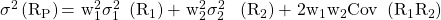Example

Continuing with our example, the variance of the portfolio is

• Weight of the first asset, w1 = 0.40
• Weight of the second asset, w2 = 0.60
• Standard deviation of first asset = 0.0357
• Standard deviation of second asset = 0.0424
• Covariance between the two assets = 0.0015

Variance of the portfolio = 0.42 x 0.03572 + 0.62 x 0.04242 + 2 x 0.4 x 0.6 x 0.0015 = 0.00157

Standard deviation of the portfolio =